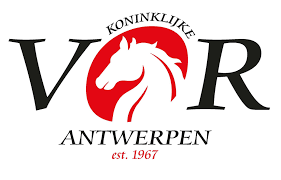OFFICIAL RESULTS LIST

## 04 125cm klasse 6 KT Barema A - 2 fazen speciaal - 274.2.5

Result list: 16 competitors in this competition. Last updated at 17:44 hrs (29.03.2019)

Rank
Nation
Competitor
CNR
Horse
 Phase 1Fehler Phase 2Fehler TotalFehler Zeit
1
 0 0 = 0.00 41.69
2
WALTER VAN MASSENHOVEN,
 0 0 = 0.00 46.22
3
 0 0 = 0.00 49.17
4
 0 0 = 0.00 50.28
5
 0 0 = 0.00 51.7
6
 0 2 = 2.00 61.95
7
 0 3 = 3.00 62.55
8
 4 0 = 4.00 53.71
9
 0 6 = 6.00 60.04
10
 8 1 = 9.00 54.57
11
 4 6 = 10.00 61.43
12
 4 7 = 11.00 62.33
13
 0 12 = 12.00 44.94
14
 0 15 = 15.00 80.17
15
 21 3 = 24.00 62.08
 0 4 eliminated